# Test Prep Plan - Take a practice test

Take this practice test to check your existing knowledge of the course material. We'll review your answers and create a Test Prep Plan for you based on your results.
How Test Prep Plans work
1
2Based on your results, we'll create a customized Test Prep Plan just for you!
3Study smarter
Study more effectively: skip concepts you already know and focus on what you still need to learn.

# Big Ideas Math 8th Grade Chapter 3: Angles & Triangles Chapter Exam

Exam Instructions:

Choose your answers to the questions and click 'Next' to see the next set of questions. You can skip questions if you would like and come back to them later with the yellow "Go To First Skipped Question" button. When you have completed the practice exam, a green submit button will appear. Click it to see your results. Good luck!

### Page 1

#### Question 3 3. If angle a is 68 degrees and angle b is 37 degrees, what is the measure of angle c?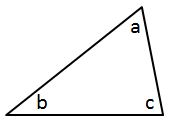### Page 2

#### Question 6 6. Which line is the transversal?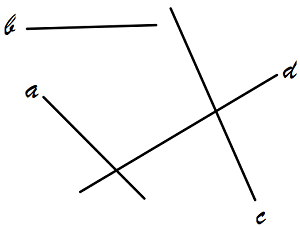#### Question 7 7. Given triangles DAR and KMR. What is the value of y?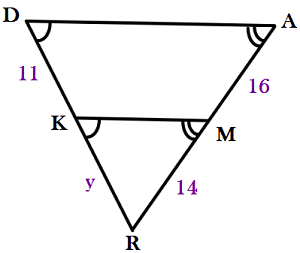### Page 3

#### Question 12 12. If angle a is 66 degrees and angle b is 54 degrees, what is the measure of angle d?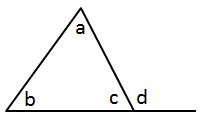#### Question 13 13. If angle a is 60 degrees, what is the measure of angle b?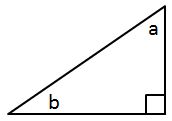#### Question 15 15. If angle a is 30 degrees and angle d is 70 degrees, what is the measure of angle b?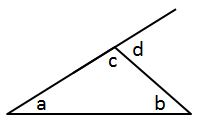### Page 4

#### Question 17 17. If angle a is 80 degrees, what is the measure of angle d?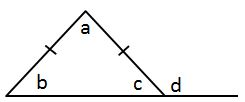#### Question 18 18. Which two lines are parallel?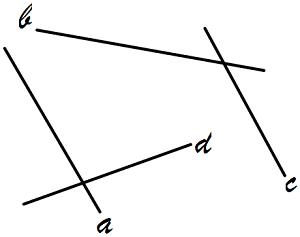#### Question 19 19. Triangle HYV and triangle AYB are similar by the AA similarity theorem. What is the value of x?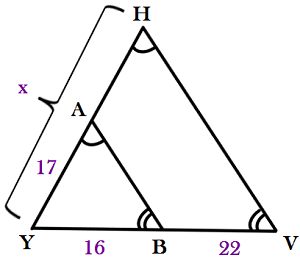### Page 5

#### Question 21 21. Study the given diagram. Find the value of x, the distance between T and E.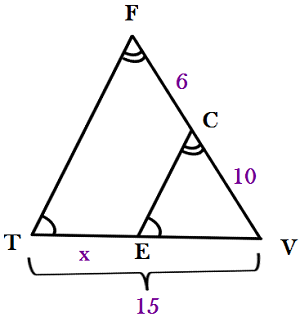#### Big Ideas Math 8th Grade Chapter 3: Angles & Triangles Chapter Exam Instructions

Choose your answers to the questions and click 'Next' to see the next set of questions. You can skip questions if you would like and come back to them later with the yellow "Go To First Skipped Question" button. When you have completed the practice exam, a green submit button will appear. Click it to see your results. Good luck!

Support